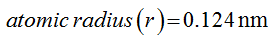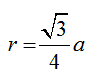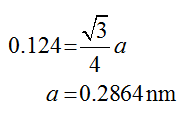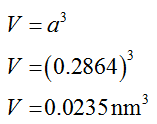# Calculate the volume of the unit cell of iron in cubic meters, given that iron has a body-center cubic crystal structure and an atomic radius of 0.124 nm.

Question
2 views

Calculate the volume of the unit cell of iron in cubic meters, given that iron has a body-center cubic crystal structure and an atomic radius of 0.124 nm.

check_circle

Step 1

Given data:Step 2

For B.C.C structure:Substituting value in the formula to find a:Step 3

Calculating volume of a given unit cell using the following formula:...

### Want to see the full answer?

See Solution

#### Want to see this answer and more?

Solutions are written by subject experts who are available 24/7. Questions are typically answered within 1 hour.*

See Solution
*Response times may vary by subject and question.
Tagged in